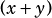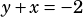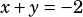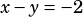y -x =2 and y + x= -2 ,Then ( x+y)(x-y)=?

We want the product ofand. We’re given that, so we know we can plug inthere.

We need to be careful on the other part. We’re given that, and we need to use that to find the value of. Let’s do it by multiplying each side by:There, so now we know thatand. Therefore,.